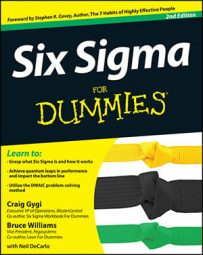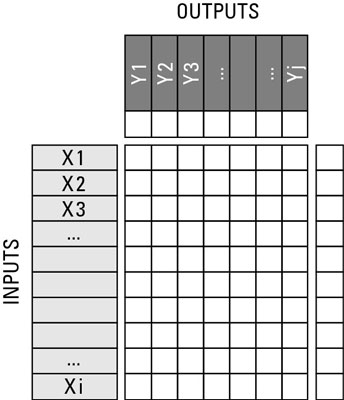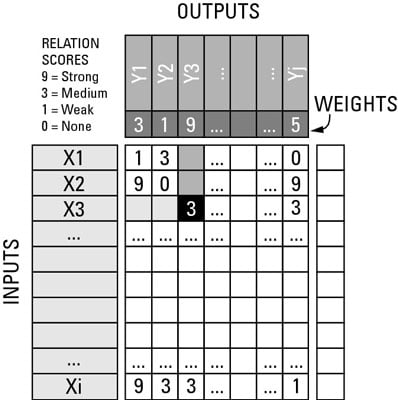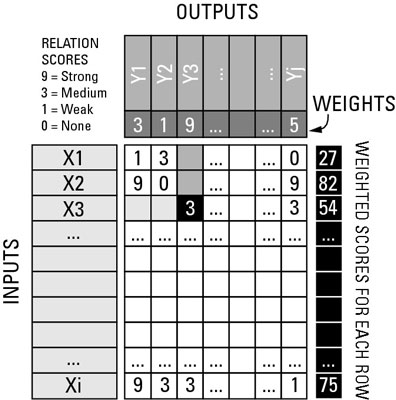##### Six Sigma For DummiesA cause-and-effect matrix — sometimes called a C&E matrix for short — helps you discover which factors affect the outcomes of your Six Sigma initiative. It provides a way of mapping out how value is transmitted from the input factors of your system (the Xs) to the process or product outputs (the Ys). With these relationships visible and quantified, you can readily discover the most-influential factors contributing to value.

## Lay out the matrixThe method employed in a C&E matrix may look familiar because it’s the basis for many familiar ranking and decision-making tools. The C&E method starts by listing all the possible input factors (the Xs) as individual rows of the matrix.

This listing of inputs should come from a previously completed process flow map or value stream map or a fishbone diagram. Using these tools before doing a C&E matrix allows you to make a comprehensive list of potential input factors without the limitations of bias or opinion.

Next, list the multiple outputs (the Ys) of the process or product across the columns of the matrix. Sometimes you have only a single output, but you usually have to simultaneously achieve several outputs, such as a performance level, a cost target, and a maximum cycle time.

With the inputs placed on the rows and the outputs listed as column headings, the C&E matrix looks like this.Next up is the relationship score — the heart of the C&E matrix. Analyze and quantify the relationships between each listed input and each output by placing a relationship score (on a scale of 0 to 9) at the matrix intersection of each row and column. Strong cause-effect relationships are scored as 9s; moderate cause-effect relationships get 3s; weak relationships are 1s; and having no relationship means a score of 0.

For each matrix row-column intersection, ask yourself whether the associated input affects the level of variation in the associated output. Then place the appropriate score in the matrix cell.

The intersection of X3 and Y3 is highlighted in black to illustrate how each row-column intersection is considered individually to score how strong of a relationship exists between the corresponding X and Y. In this example, it’s a 3 — a moderate correlation.

## Figure the final scoreWith the scoring of the matrix completed, summarize the results by calculating the weighted score for each row. Go to each row and multiply the first matrix cell score by the first column weight; add that result to the second matrix cell score and multiply the result by the second column weight, and so on, adding up the weighted scores for the entire row.

Place this weighted row sum in the far right column of the C&E matrix.

The final step is to apply Pareto analysis to the scores for each row. Those rows with high scores are the ones that indicate important, high-leverage input factors. You can effectively ignore the low scores from further consideration.